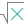#SQRTPI (PQL - xl)

This function returns the positive square root of a multiple of pi (p)

• Library: PQL \ Spreadsheet \ Math & Trig
• Compatibility: Any content (regardless of data source) in the Tabulate spreadsheet module
• Solve: This function can be used with the Solve plug-in: both as part of the objective function and constraint functions

#### Syntax

SQRTPI(number)

##### Function Arguments
 Name Description Type Optional number Specify the number of multiples of pi (p) of which to calculate the square root Number